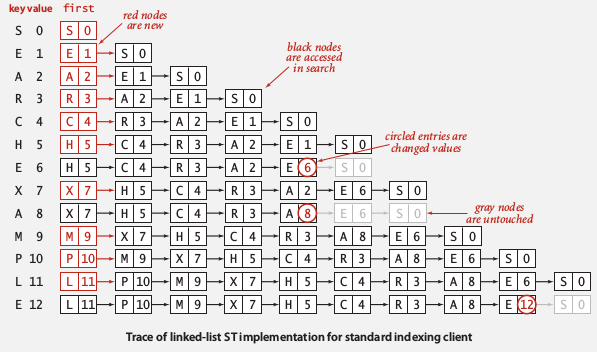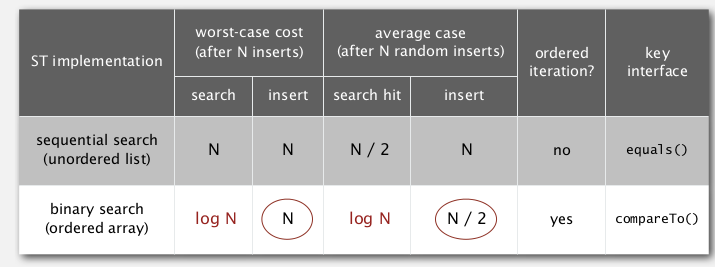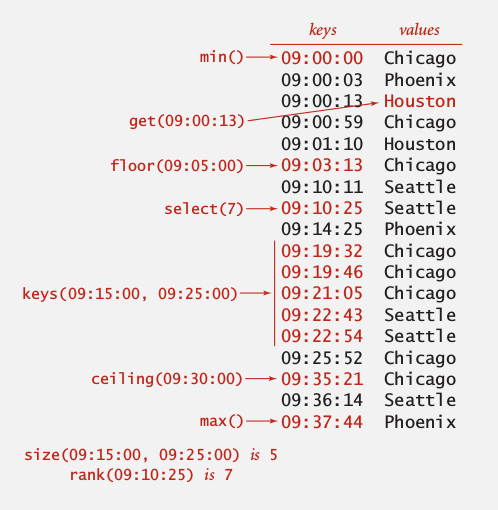[TOC]

# 1. Symbol Table API

key-value pair abstraction

• insert a value with a key
• given a key, search for its value

## Association array abstraction

Associate a value to a key — generalized array: a[key]=val.

```public class ST<Key, Value>{
void put(Key k, Value v);//remove key if value=null
Value get(Key k);//return null if key is absent
void delete(Key k);
boolean contains(Key k);
boolean isEmpty();
int size();
Iterable<Key> keys();//better to return an ordered sequence of keys
}
```

conventions:

• values are not null
• get() returns null if key not present
• put() can overwrite older value

→ some one-line implementations:

• contains: `return get(k)!=null;`
• delete: `put(k, null);`

Assume keys to be comparable: `class ST<Key implements Comparable<Key>, Value>`— can thus use `compareTo()` method.
Else → we can only use the `equals()` method...
Be careful when implementing the equals method: 坑不少...# 2. Elementary implementations

naive implementations

## using unordered linked list

ListNode{key, value, next}

• search: scan through all keys ~N
• insert: scan through, if not found, add to front ~N## using ordered array

using 2 arrays: keys[] (sorted), vals[]
⇒ can improve performance by binary search

search operation
write a function rank() that returns the number of keys < k searched.

```private int rank(Key k){
int lo=0, hi=keys.length-1;
while(hi>=lo){
int mid = lo + (hi-lo)/2;
int cmp = keys[mid].compareTo(keys[k]);
if(cmp==0) return mid;
else if(cmp>0) hi = mid-1;
else lo = mid+1;
}
return lo;
}
```

Using rank() to implement the get() method:

```public Value get(Key k){
int rk = rank(k);
if(rk<N && keys[rk].compareTo(k)==0) return vals[rk];
return null;
}
```

insert operation
Like insertion sort, time complexity is ~N for each insert.

summery:# 3. Ordered Opeartions

When keys are comparable ⇒ provide more functionalities in the API.
for example:

• `min()/max()`: min/max key
• `deleteMin()/deleteMax()`
• `floor(Key k)/ceiling(Key k)`: largest key <=k / smallest key >=k
• `rank(Key k)`: nb of keys < key
• `select(int i)`: key with rank=i
• `Iterator<Key> keys(lo, hi)`: iterates through [lo, hi]#### Part 9 of series «Algorithms Princeton MOOC I»：

comments powered by Disqus